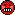## Alternative CI for BE decision [Power / Sample Size]

Dear zizou,

all you have written about CI(s) is more or less correct.
But it's a misunderstanding.

The basic test to use for the BE decision is TOST with alpha = 0.05 by convention. And this is operationally identical to the interval inclusion rule, conventionally using an ordinary 1-2*alpha CI.

The Berger, Hsu alternative CI+ is another CI (an 1-alpha CI) constructed based on TOST and gives the same result if used as decision rule for stating BE.

Code to show this via simulations:
power.sim2x2 <- function(CV, GMR, n, nsims=1E5, alpha=0.05, lBEL=0.8, uBEL=1.25,                          CItype=c("usual", "alternative"), setseed=TRUE, details=FALSE) {   ptm <- proc.time()   if(setseed) set.seed(123456)   # check CItype   CI <- match.arg(CItype)   # n is total if given as simple number   # to be correct n must then be even!   if (length(n)==1) {     nsum <- n     fact <- 2/n   }   if (length(n)==2) {     nsum <- sum(n)     fact <- 0.5*sum(1/n)   }   mse    <- log(1.0 + CV^2)   df     <- nsum-2   tval   <- qt(1-alpha,df)   # Attention! With nsims=1E8 memory of my machine (4 GB) is too low   # Thus work in chunks of 1E6 if nsims>1E6.   chunks <- 1   ns     <- nsims   if (nsims>1e6) {     chunks <- trunc(nsims/1E6)     ns     <- 1E6     if (chunks*1e6!=nsims){       nsims <- chunks*1e6       warning("nsims truncated to", nsims)     }   }   BEcount <- 0   sdm     <- sqrt(fact*mse)   mlog    <- log(GMR)   for (i in 1:chunks)   {     # simulate sample mean via its normal distribution     means  <- rnorm(ns, mean=mlog, sd=sdm)     # simulate sample mse via chi-square distribution of df*mses/mse     mses   <- mse*rchisq(ns,df)/df     hw     <- tval*sqrt(fact*mses)     lCL <- means - hw     uCL <- means + hw     if(CI=="alternative"){       # CI+, i.e. expand CI to contain GMR=1       lCL <- ifelse(lCL<0, lCL, 0)       uCL <- ifelse(uCL>0, uCL, 0)     }     # point  <- exp(means)     lCL    <- exp(lCL)     uCL    <- exp(uCL)     # standard check if CI of GMR (usual or alternative)     # is in 0.8-1.25 (without rounding CI limits)     BE     <- (lBEL<=lCL & uCL<=uBEL)     BEcount <- BEcount + sum(BE)   }   if (details) {     cat(nsims,"sims. Time elapsed (sec):\n")     print(proc.time()-ptm)   }   BEcount/nsims } 

And now try:
power.sim2x2(CV=0.2, n=24, GMR=0.95, CItype="usual")  0.89572 power.sim2x2(CV=0.2, n=24, GMR=0.95, CItype="alternative")  0.89572
Quod errat demonstrandum. At least for CV=0.2, n=24 and GMR=0.95.

BTW: It's not necessary to rely on simulations. The EMA BSWP f.i. doesn't like simulations. You may consider different cases for lCL, uCL to show that the alternative CI+ gives always the same decision as the usual 1-2*alpha CI.
F.i.:
If the usual CI is within the acceptance range (with upper CL below 1) -> decide BE
Then the alternative CI with upper CL set to 1 is also in the acceptance range -> decide BE.
And so on for other constellations.

Regards,

Detlew

### Complete thread:

Admin contact
21,419 posts in 4,475 threads, 1,510 registered users;
online 11 (0 registered, 11 guests [including 2 identified bots]).
Forum time: Wednesday 02:11 UTC (Europe/Vienna)

In the Middles Ages the lingua franca of science was Latin.
Nowadays the language of science is bad English.    Anonymous

The Bioequivalence and Bioavailability Forum is hosted byIng. Helmut Schütz